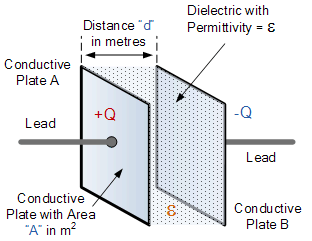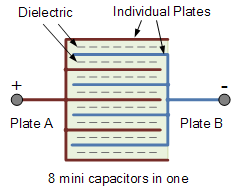# 电容器简介## 电容器的电容

### 标准电容单位

• 微法（μF1 μF= 1 / 1,000,000 = 0.000001 = 10-6 F.
• 纳法（nF1 nF = 1 / 1,000,000,000 = 0.000000001 = 10-9 F.
• 皮法（pF1 pF = 1 / 1,000,000,000,000 = 0.000000000001 = 10-12 F.

1,000 1.0 0.001
10,000 10.0 0.01
1,000,000 1,000 1.0
10,000 10.0
100,000 100
1,000,000 1,000 0.001
10,000 0.01
100,000 0.1
1,000,000 1.0

## 平行板电容器的电容## 电容示例 No1

$$C = \epsilon \cdot { A \over d}$$

$$\epsilon_0 = 8.84 \times 10^{-10}$$ $$A = 0.3 \times 0.5 m^{2} \quad and \quad d=6 \times 10^{-3}m$$ $$C = {8.84 \times 10^{-12} \times \left( 0.3 \times 0.5 \right) \over 6 \times 10^{-3}} = 0.221 nF$$

## 电容器的介电

### 复介电常数

$$\epsilon = \epsilon_{0} \times \epsilon_{r}$$

$$C = {\epsilon_{0}\epsilon_{r}A \over d}$$

$$C = {\epsilon{0}\epsilon{r}\left( n-1\right) A\over d}$$

### 多板电容器$$C = {\epsilon_{0} \epsilon_{r} \left( n-1\right) A \over d} = {\epsilon_{0} \epsilon_{r} \left( 9-1 \right) A \over d} = {\epsilon_{0} \epsilon_{r} 8 A \over d}$$

• 低损耗，高稳定性，如云母，低 K 陶瓷，聚苯乙烯。
• 中等损耗，中等稳定性，如纸张，塑料薄膜，高 K 陶瓷。
• 极化电容器，如电解电容器，钽电容器。

## 电容器简介摘要

• 表面积 - 构成电容器的两个导电板的表面积 A，面积越大，电容越大。
• 距离 - 两个板之间的距离 d，距离越小，电容越大。
• 介电材料 - 将两个板分开称为“电介质”的材料类型，电介质的介电常数越高，电容越大。

• 介电常数是介电材料的特性，并且从一种材料到另一种材料不同，使电容增加 k 倍。
• 电介质在两个板之间提供机械支撑，允许板在不接触的情况下更靠近在一起。
• 电介质的介电常数增加了电容。
• 与空气相比，电介质增加了最大工作电压。